Published 11.08.2003.
Updated 19.09.2003 with table 5.

WATER ELECTRIC GENERATOR OF HEAT

Ph. M. Kanarev. G. P. Perekoty, D.A. Bebko, A.A. Chernyavsky

E-mail: kanphil@mail.kuban.ru

Abstract: the measurement process analysis of energy indices of the water electric generator of heat shows that there are such design of this generator and its such operation modes when quantity of heat energy generated by water exceeds the expenses of electrical energy for this process significantly.

INTRODUCTION

In engineering practice connected with ventilation system servicing, a phenomenon of excessive thermal energy in circulating air has been found. Similar phenomenon has been registered in water circulation systems with the devices for its active cavitations. In the Russian market, three firms (Yusmar, Termovikhr and Noteka) sell cavitation water heating equipment with energy efficiency index of 150%. Soon, an air heating device with the same efficiency will be produced.

We have already shown that energy of physical vacuum taken by valence electrons of the molecules after their mechanical destruction and emitted by these electrons within the repeated fusion of the molecules is the most probable source of additional energy generated by the ventilation systems and the cavitation ones [1 - 5]. It is explained by the fact that half as much energy is spent for mechanical destruction of the molecules than for thermal destruction of these molecules. Valence electrons of the molecules being destroyed mechanically absorb energy from physical vacuum in order to restore their energy indices and emit it during the repeated fusion of these molecules.

As half as much energy is spent for mechanical destruction of the molecules than for thermal one, energy effectiveness index of such processes cannot exceed two. But if this hypothesis is correct, there is a possibility to increase energy effectiveness index of this process considerably when the molecules are destroyed electrodynamically. In this case, there is a possibility to find resonance modes of electrodynamic destruction of the molecules and to reduce energy consumption for this process considerably. Further fusion of the molecules being destroyed electrodynamically will release required quantity of energy, which will exceed considerably the energy being spent.

The results of generation of additional energy during electrodynamic destruction of water molecules have already been published [4,  6]. As in this process special pulses of current and voltage were generated and the point magnetoelectric voltmeter and ammeter were used for measurement of their mean values, it became necessary to determine if a truncation error, which led to overestimation or underestimation of a design index of efficiency, took place during the instrument measurements. In this connection, ACK-2022 electron oscillograph was used in addition when measurements were carried out. As there was no computer processing system, the oscillogram parameters of voltage and current being obtained were processed manually. The mean values of voltage and current were calculated according to the formulas: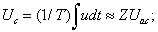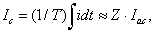where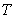is measurement period;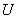is instantaneous voltage;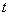is time;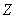is duty factor;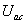is mean value of voltage pulse amplitude;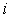is instantaneous current;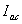is mean value of current pulse amplitude.

Duty factor was determined according to the formula:    Z =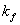/ S,  whereis pulse form factor,  S is off-duty factor:    S =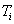/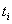,   whereis pulse follow period,is pulse length. For pulses of the exponential form and triangular one being used in the experiments, the curve form factor in the calculations is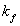= 0.5.

EXPERIMENTAL PART

The main task of the experiment was to check the hypothesis: “Electrodynamic effect on the molecules gives the possibility to form resonant modes when energy consumption for the destruction of their bonds is reduced considerably”. In order to solve this task, special experiments were carried out connected with electrodynamic destruction of chemical bonds of water molecules with electric pulses of various frequencies. The diagram of the installation used for experimental investigations is shown in Fig. 1; the photo of the experimental generator of heat is shown in Fig. 2.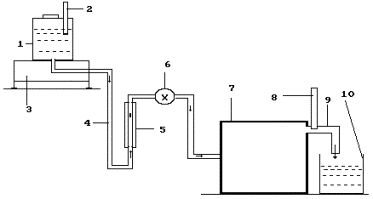Fig. 6. Diagram of the experimental installation: 1 - reservoir for solution; 2- thermometer; 3- electronic scales; 4 - solution feed duct; 5- rotameter; 6- solution feed regulator; 7-a special thin plasma generator is in the process of patenting; 8 - thermometer; 9- heated solution discharge; 10- inlet reservoir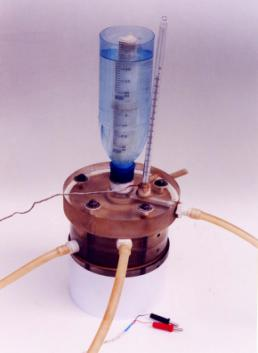Fig. 2. Photo of generator heart

The results of the experiments are given in Figs  3  -  6 and in Tables 1  -  4.

The oscillogram of voltage pulses is given in Fig.  3; the oscillogram of current pulses influencing the generator of heat in one of the experiments carried out with pulse frequency of nearly 100 Hz is given in Fig. 4. As it is clear from the oscillograms, the pulses of both voltage and current have an exponential form being close to the triangular one with a sharp edge and a shallow declination. The design duty factor for these pulses is Z » 0.039. Mean amplitude of voltage pulses is equal to power supply voltage of the pulse generator: 250 V. Thus, a mean component of voltage pulses being brought to the generator of heat is equal to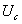= 0.039 х 250 = 9.75 V. In this experiment, voltmeter readings were 10.0 V.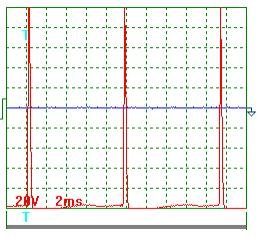Fig. 3.  Oscillogram of power supply voltage pulses at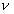» 100 Hz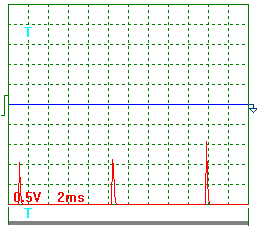Fig. 4. Oscillogram of current pulses via the generator of heat at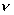» 100 Hz

A current pulse oscillogram in this experiment is shown in Fig. 4. Current was measured as voltage drop at a measuring resistor with resistance of  0.1 ohm included into the supply circuit of generator of heat. As it is clear, mean amplitude of current pulses is 1.3 / 0.1 = 13 А, and mean component value is equal to: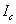= 0.039 х 13 =  0.51 А. During measurements, the ammeter showed current of   0.50 A.

On the basis of the oscillographic measurement data, mean value of electric power has proved to be Р = 9.8х0.51 = 5.0 W. The experiment lasted for 300 seconds. Thus, electric energy of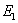=5.0 х 300 = 1500 J = 1.5 kJ entered the generator of heat. During this period, it heated 0.55 kg of solution by 12 degrees. Energy value of this heat was equal to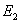=4.19х0.55х12=27.65 kJ. Efficiency index of energy process was  К =  Е2 / Е1 = 27.65 / 1.5 = 18.43, or 1843% (s. Table 2).  It corresponds (with accuracy of up to 5% being characteristic of oscillographic check) to energy efficiency index being determined with the help of the voltmeter and the ammeter (s. Table 1).

Table 1

Experimental indices of the water electric generator of heat  with electric pulse frequency of nearly 100 Hz with the measurements of mean values of voltages and current with the help of the voltmeter and the ammeter

 Indices Mean 1. Mass of the solution, which has passed through the generator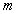,  kg. 0.55 2. Temperature of solution at the input of the generator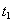, degrees 26.00 3. Temperature of the solution at the output of the generator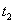, degrees 38.00 4. Temperature difference of the solution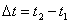, degrees 12.00 5. Durability of the experiment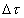, s 300.00 6. Reading of voltmeter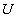, V 10.50 7. Reading of ammeter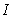, A 0.50 8. Electric power consumption,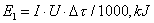1.57 9 – power spent for heating of the solution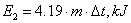27.65 10 – reactor efficiency index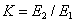17.61

Table 2

Experimental indices of the water electric generator of heat  with electric pulse frequency of nearly 100 Hz with the measurements of mean values of voltages and current with the help of the electronic oscillograph

 Indices Mean 1. Mass of the solution, which has passed through the generator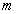,  kg. 0.55 2. Temperature of solution at the input of the generator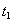, degrees 26.00 3. Temperature of the solution at the output of the generator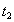, degrees 38.00 4. Temperature difference of the solution, degrees 12.00 5. Durability of the experiment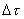, s 300.00 6. Mean value of voltage, V 9.75 7. Mean value of current, A 0.51 8. Power consumption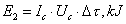1.50 9. Power spent for heating of the solution,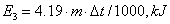27.65 10. Generator  efficiency index K= E3/ E2 18.43

In Fig. 5, the oscillogram of voltage pulses is given. In Fig. 6, the oscillogram of current pulses being registered during another experiment with pulse frequency of nearly 300 Hz is given. According to these oscillograms, the duty factor calculation has given the result of Z = 0.11. With mean values of amplitudes of pulses of voltage and current being equal to 250 V and 10.6 A, respectively, the mean components of voltage and current arriving into the generator of heat have been:= 0,11 х 250 = 27.5 V;= 0.11 х 10.6 = 1.17 A. According to the readings of the voltmeter and the ammeter, mean values of voltage and current were 25.0 V and 1.25 A in this experiment. In this connection, mean value of electric power supplied to the generator of heat was 27.5 х 1.17 = 32.18 W according to the data of the oscillographic measurements and 25 х 1.25 = 31.25 W according to the data of the pointer indicators. Divergence in this methods of mean power determination did not exceed 5% as well.

The energy efficiency calculation results of the generators of the heat for both methods of measurement with pulse frequency of nearly 300 Hz are given in Table 3 and 4. They are close in their values as well.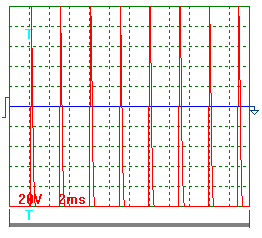Fig. 5. Oscillogram of supply voltage pulses at» 300 Hz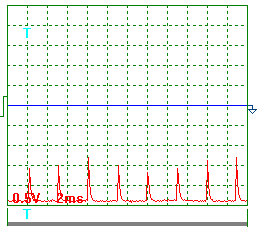Fig. 6. Oscillogram of current pulses via the generator of heat at» 300 Hz

Table 3

Experimental indices of the water electric generator of heat  with electric pulse frequency of nearly 300 Hz with the measurements of mean values of voltages and current with the help of the voltmeter and the ammeter

 Indices Mean 1. Mass of the solution, which has passed through the generator,  kg. 0.41 2. Temperature of solution at the input of the generator, degrees 26.00 3. Temperature of the solution at the output of the generator, degrees 76.00 4. Temperature difference of the solution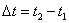, degrees 50.00 5. Durability of the experiment, s 300.00 6. Reading of voltmeter, V 25.00 7. Reading of ammeter, A 1.25 8. Electric power consumption,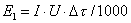, kJ 9.38 9. Power spent for heating of the solution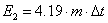, kJ 85.90 10. Generator  efficiency index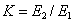9.16

Table 4

Experimental indices of the water electric generator of heat  with electric pulse frequency of nearly 300 Hz with the measurements of mean values of voltages and current with the help of the electronic oscillograph

 Indices Mean 1. Mass of the solution, which has passed through the generator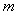,  kg. 0.41 2. Temperature of solution at the input of the generator, degrees 26.00 3. Temperature of the solution at the output of the generator, degrees 76.00 4. Temperature difference of the solution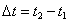, degrees 50.00 5. Durability of the experiment, s 300.00 6. Mean value of voltage, V 27.5 7. Mean value of current, A 1.17 8. Power consumption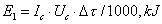9.65 9. Power spent for heating of the solution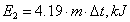85.89 10. Generator  efficiency index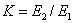8.90

Table 5

PROTOCOL  OF CONTROL TEST

 Indices 1 2 3 Mean 1. Mass of the solution, which has passed through the generator,  kg. 0.384 0.372 0.378 0.378 2. Temperature of solution at the input of the generator, degrees 27 27 27 27 3. Temperature of the solution at the output of the generator, degrees 85 86 86 85.3 4. Temperature difference of the solution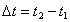, degrees 58 59 59 58.7 5. Durability of the experiment, s 300 300 300 300 6. Mean value of voltage, V 5.5 5.5 5.5 5.5 7. Mean value of current, A 2.6 2.6 2.6 2.60 8. Power consumption4.29 4.29 4.29 4.29 9. Power spent for heating of the solution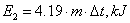93.32 91.96 93.44 92.91 10. Generator  efficiency index21.75 21.44 21.78 21.66

Thus, it is possible to consider that an experimental check of energy efficiency of the water electric generator of heat with the help of two methods gives practically the same results and confirms the above-mentioned hypothesis concerning the possibility of additional energy production in the processes being considered [6, 7]. It can be noted that as during measurements the pointer instruments of high class of accuracy of 0.2 have been used (relative conventional gauging error does not exceed 0.2%) and oscillographic measurement accuracy is much lower (usually nearly 5%), the results given in Tables 1 and 3 can be considered more accurate.

Commercial efficiency of the water electric generator of heat will depend on pulse generator economies. As efficiency of powerful pulse generators can be near unit, energy efficiency should not differ greatly from the data being obtained during laboratory investigations for the industrial-scale plants with the use of the generators of heat being considered.

CONCLUSION

The analysis of energy balance of the molecules with covalent bonds shows the possibility of formation of additional thermal energy with the energy efficiency index, which exceeds unity greatly, and the experiments confirm this hypothesis earnestly.

Simplicity and hundred per cent reproducibility of the experiments being described open a prospect for quick commercialization of the water electric generator of heat.

REFERENCES

1. Kanarev Ph.M. The Foundation of  Physchemistry of  Microworld – Krasnodar: the Kuban State Agrarian University, 2002. – 320 pages. (In Russian).

2. Kanarev Ph.M. The Foundation of  Physchemistry of  Microworld /the second edition/. – Krasnodar: the Kuban State Agrarian University, 2003. – 330 pages. (In Russian)  http://www.ikar.udm.ru/sb28-2.htm

3. Kanarev Ph.M. The Foundation of  Physchemistry of  Microworld. The second edition. (In English). http://book.physchemistry.innoplaza.net

4. Kanarev Ph.M. Energy Balance of Fusion Process of Oxygen, Hydrogen and Water Molecules. New Energy Technologies. 2003, No. 3 (12), pages 58-62.  (In Russian)

5. Kanarev Ph.M. Global Energy. New Energy Technologies. 2003, No. 3 (12), pages 56-57. (In Russian).

6. Kanarev Ph.M. Energy Balance of Fusion Process of Oxygen, Hydrogen and Water Molecules. New Energy Technologies. 2003, Issue No. 3 (12), pages 58-62. (In English).

7. Kanarev Ph.M. Global Energy. New Energy Technologies. 2003, Issue No. 3 (12), 2003, pages 56-57. (In English).

Webmaster: J. Hartikka

Water Electric Generator of Heat:

http://Kanarev.heatgenerator.innoplaza.net

<< Kanarev´s Page